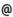## "Babes-Bolyai" University of Cluj-Napoca Faculty of Mathematics and Computer Science

 Mathematical Analysis
 Code Semes-ter Hours: C+S+L Type Section MMA0002 1 3+2+0 compulsory Informatică
 Teaching Staff in Charge
 Prof. MURESAN Marian, Ph.D.,  mmarianmath.ubbcluj.roLect. TRIF Tiberiu Vasile, Ph.D.,  ttrifmath.ubbcluj.roAssoc.Prof. SÁNDOR Jozsef, Ph.D.,  jsandormath.ubbcluj.roProf. KASSAY Gabor, Ph.D.,  kassaymath.ubbcluj.ro
 Aims Getting to know the topology of IRn and the basic notions and results concerning the multivariable differential calculus.
 Content 1. Real numbers. Sequences and series of real numbers. 2. Topology of IRn space. 3. Limites of real and vectorial functions of one and several variables. 4. Continuity and uniform continuity of real and vectorial functions of one and several variables 5. Differential calculus on R. The derivative and the differential of a real function. Operations with differentiable functions. Differentiability of the compound function and of the inverse function. Mean value theorems (Fermat, Rolle, Cauchy, Lagrange). L'Hospital rule. Higher order derivatives. The characterization of convexity by the sign of derivatives. Taylor formula. Local extrem points of a real function and their characterization. 6. Partial derivatives and differentiability of real and vector functions of several variables; Taylor's formula for real function of several variables. 7. The inverse function theorem; differentiable implicit functions. 8. Optimality conditions; optimization problems having equations as constraints. The basic notions and results concerning the multivariable differential and integral calculus are presented. The main topics included are: differentiability of vector functions of vector variable, mean value theorems for differentiable functions, partial derivatives, optimality conditions, the inverse function theorem, differentiable implicit functions, optimization problems having equations as constraints
 References 1. ANDRICA D., DUCA I.D., PURDEA I., POP I.: Matematica de baza. Cluj-Napoca, Editura Studium, 2002. 2. BALAZS M., KOLUMBAN I.: Analiza matematica. Curs litografiat, Facultatea de Matematica, Univ. "Babes-Bolyai". 3. BRECKNER W. W.: Analiza matematica. Topologia spatiului Rn. Cluj-Napoca, Universitatea, 1985. 4. COBZAS ST.: Analiza matematica (Calcul diferential). Cluj-Napoca, Presa Universitara Clujeana, 1998. 5. LUPSA L., BLAGA R.L. Elemente de analiza matematica si teoria campului. Partea I. Bistrita, Editura George Cosbuc, 2001. 6. LUPSA L., BLAGA R.L. Elemente de analiza matematica si teoria campului. Partea I. Cluj-Napoca, Ed. RISOPRINT, 2002. 7. MARUSCIAC I.: Analiza matematica. I, II. Cluj-Napoca, Universitatea "Babes-Bolyai", 1980. 8. FIHTENHOLT G. M.: Curs de calcul diferential si integral. Vol. I, II. Bucuresti, Editura Tehnica, 1965. 9. TRIF T.: Probleme de calcul diferential si integral in IRn. Cluj-Napoca Ed. Casa Cartii de stiinta, 2003. 10. MEGAN M. Basele analizei matematice. Timisoara Ed. BIT, vol I - III, 2000, 2001, 2002.
 Assessment Exam.
 Links: Syllabus for all subjects Romanian version for this subject Rtf format for this subject